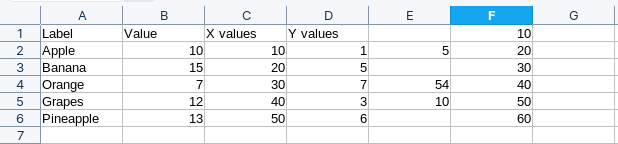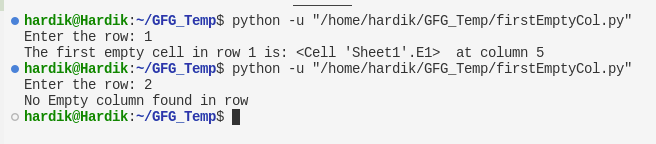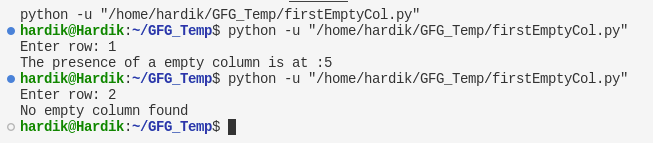GFG App
Open AppBrowser
Continue

# Finding First Empty Column in an Excel Sheet using Python

Excel is a spreadsheet in a computer application that is designed to add, display, analyze, organize, and manipulate data arranged in rows and columns. It is the most popular application for accounting, analytics, data presentation, etc. In this article, we will learn how to store user input in an excel sheet using Python. Methods used for taking user input in an excel sheet using Python.

In this tutorial we are going to use the below dataset you can download it from here:### Finding First Empty Column using openpyxl module

openpyxl module: Openpyxl is a Python library for reading and writing Excel (with extension xlsx/xlsm/xltx/xltm) files. The openpyxl module allows Python programs to read and modify Excel files.

Step 1: Import openpyxl module

`import openpyxl`

load_workbook() function opens the given file and returns the workbook

`wb = openpyxl.load_workbook('filename.xlsx')`

Step 3: Specify the sheet in which we are going to read the data. Since we have only one sheet, we can just use the active keyword to use the currently active sheet to enter the data.

`sheet = wb.activate`

In case you have multiple sheets, you have to mention the name of the worksheet as follows:

`sheet = wb["SheetName"]`

Step 4: Take the input row in which you want to search the empty column

`row = int(input("Enter the row: "))`

Step 5: Set a flag that will tell us if is there an empty column present in the row or not

`flag = 1`

Step 6: Find whether there exists an empty column in the row or not. Iterate each column in the row and check whether the cell value is None or not.

## Python3

 `import` `openpyxl ` `wb ``=` `openpyxl.load_workbook(``'data.xlsx'``) ` `sheet ``=` `wb.active ` `row ``=` `int``(``input``(``"Enter the row: "``)) ` `flag ``=` `1` `for` `colNum ``in` `range``(``1``, sheet.max_column``+``1``): ` `    ``if` `sheet.cell(row``=``row, column``=``colNum).value ``=``=` `None``: ` `        ``flag ``=` `0` `        ``print``(f``"The first empty cell in row {row} is:"``,sheet.cell ` `              ``(row``=``1``, column``=``colNum),f``" at column {colNum}"``) ` `        ``break` `if` `flag: ``print``(``'No Empty column found in row'``)`

Output:### Finding the First Empty Column using xlrd module

The xlrd library is for reading data and formatting information from Excel files in the historical .xls format. This module only supports files having .xls extension if you want to work on .xlsx file, you can install the 1.2.0 version of the module.

Step 1: Import xlrd module

`import xlrd`

Step 2: Open the workbook

• Use the open_wordkbook() function to open the given file and returns the workbook
`wb = xlrd.open_workbook('filename.xlsx')`

Step 3: Specify the sheet in which we are going to enter the data

`sheet = wb.sheet_by_index(0)`

Step 4: Take the input row in which you want to search the empty column. We will subtract 1 from the user input since we are working on 0-based indexing

`row = int(input("Enter the row: "))-1`

Step 5: Set a flag that will tell us if is there an empty column present in the row or not

`flag = 0`

Step 6: Find whether there exists an empty column in the row or not. Iterate each column in the row and check whether the cell value is Empty or not.

## Python3

 `import` `xlrd ` `wb ``=` `xlrd.open_workbook(``"data.xlsx"``) ` `sheet ``=` `wb.sheet_by_index(``0``) ` `flag ``=` `0` `row ``=` `int``(``input``(``"Enter row: "``))``-``1` `for` `col ``in` `range` `(sheet.ncols) : ` `    ``if` `sheet.cell(row,col).value ``=``=` `'': ` `        ``column ``=` `col ` `        ``flag ``=` `1` `        ``break` `if` `flag: ``print``(f``'The presence of a empty column is at :{col+1}'``) ` `else``: ``print``(``"No empty column found"``)`

Output:My Personal Notes arrow_drop_up